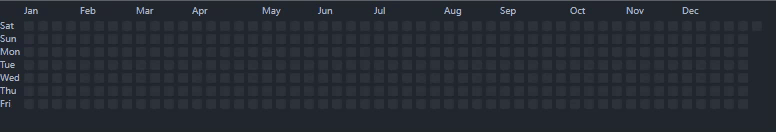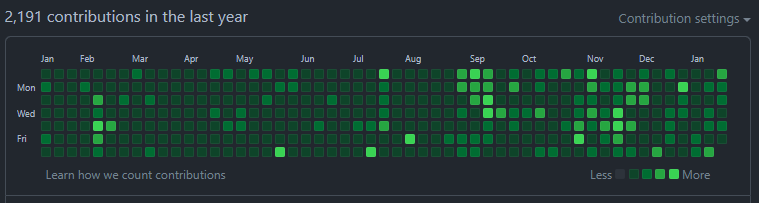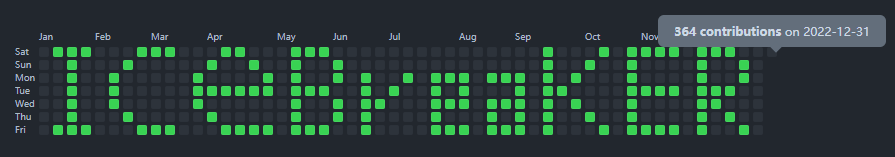# d3-selection 入门篇（1）,制作响应式 Github ContributionCalendar

d3
ContributionCalendar
d3-selection
reactive# d3-selection 入门篇（1）,制作响应式 Github ContributionCalendar

## 写一个简单的Demo## 开始撰写

### 流程式写法

``````// 一列 7 个正方形
const colItemCount = 7
// 创建一个 svg ，里面一个 g
const wrapper = d3
.select(boxRef.value as HTMLDivElement)
.append('svg')
.attr('width', 722)
.attr('height', 112)
.append('g')
.attr('transform', 'translate(10, 20)')

let colIndex = -1
let g: d3.Selection<SVGGElement, unknown, null, undefined>
// 一年有 365 个日出，我送你 365 个祝福
for (let i = 0; i < 365; i++) {
// y 轴的索引
const yIdx = i % colItemCount
if (yIdx === 0) {
// 当第一个的时候，指针指到第一列,添加一个 g 对 rect 进行包裹
colIndex++
g = wrapper
.append('g')
.attr('transform', `translate(\${colIndex * 14}, 0)`)
}
// 正方形
g!
.append('rect')
.attr('width', 10)
.attr('height', 10)
.attr('x', 14)
// y轴偏移
.attr('y', yIdx * 13)
.attr('rx', 2)
.attr('ry', 2)
.attr('data-level', i % 4)
// 添加 class ， attr('class','ContributionCalendar-day')也行
.classed('ContributionCalendar-day', true)
.each(function () {
// 鼠标移上去显示 Popper
})
}
// 添加 x 轴
// 添加 y 轴
``````

1. 假如这是一个 `render` 方法，当我们改变其中的值后，再次调用重新渲染，它会再添加一个 `svg` 并进行一些 `dom` 操作。显然此时把一些 `selection` 缓存起来是比较好的选择。
2. `function` 虽然有拆分，但是变量之间的耦合度太高。

### 数据驱动式写法

`d3-selection` 中除了一些选择赋值这些基础的dom操作API，另一个极其重要的就是要掌握 `Joining Data`，即:

• `selection.data([data[, key]])`
• `selection.join(enter[, update][, exit])`

`data` 在很多场景下极其有用，比如我们处理自引用树状结构时，就要用它结合 `d3-hierarchy` 一起使用。

`join` 本身就是 `enter``exit`，在回调部分通过数据的变化对选择集合进行归类，并执行自定义回调。

`datum` 则可以从现有的数据集合中抽取更改链式调用的数据源。

#### 准备数据源

``````type MatrixItem = {
id: string
index: number
level: number
count: number
date: string
}
let matrix: MatrixItem[][]
// then fill the matrix
matrix = fillMatrix(seed)
``````

``````// render 时接管 svg 的宽度
const width = Math.floor(matrix.length + 1) * 14 + 24
svg.attr('width', width).attr('height', height)

wrapper
.selectAll('g')
// 注入数据
.data(data)
// 二维数组 第一层，选中 MatrixItem[] , enter -> g
.join('g')
.attr('transform', function (d, i, g) {
return `translate(\${(i + 1) * 14}, 0)`
})
.selectAll('rect')
// 二维数组 第二层，选中 MatrixItem
.data((d) => d)
.join(
// enter
(enter) => {
// 添加小方块
return enter
.append('rect')
.attr('width', 10)
.attr('height', 10)
.attr('x', 0)
.attr('y', function (d, i) {
return i * 13
})
.attr('rx', 2)
.attr('ry', 2)
.attr('data-count', function (d, i) {
return d.count
})
.attr('data-date', function (d, i) {
return d.date
})
.classed('ContributionCalendar-day', true)
.each(function (d, i, g) {
})
.attr('data-level', function (d, i) {
return d.level
})
},
// update 时 level更改，进行变色
(update) =>
update.attr('data-level', function (d, i) {
d.level = (d.level + seed) % 4
return d.level
}),
// exit 时，移除 dom
(exit) => exit.remove()
)
``````D3’s data join lets you specify exactly what happens to the DOM as data changes. This makes it fast—you can minimize DOM operations—and expressive—you can animate enter, update and exit separately. Yet power comes at a cost: the data join’s generality makes it hard to learn and easy to forget.

``````update = new Selection(update, parents);
update._enter = enter;
update._exit = exit;
return update;
``````

## 附录

@d3/selection-join

Thinking With Joins

selection.data source

selection.join source

Demo folder